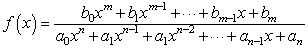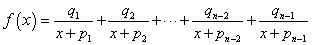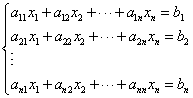# Math tools

This page is a web application that solve an equation of higher degree, simultaneous equations, partial fraction decomposition. Transfer function analysis and design tool

## Equation of higher degree

a0xn+a1xn-1+ ••• +an-1x+an=0

### Example

x4-10x3+35x2-50x+24=0

The following form is an example to input G(s). Use the right form to simulate.

(maximum n=12)
a=

## Partial fraction decomposition↓Transient analysis condition is nm. maximum n=12

### Example

 f(x)= 10x3+80x2+200x+154 x4+10x3+35x2+50x+24
The following form is an example to input. Use the right form to calculate.

b=
a=
b=
a=

## Simultaneous equations### Example

2x1 -7x2+6x3=4
-5x1      -x3=1
4x1+3x2    = -9
The following form is an example to input. Use the right form to calculate.

 2 -7 6 -5 0 -1 4 3 0 4 1 -9
maximum n=8

## Scientific Calculator

### Example

e-(1e3/100e-6) cos 10e3/(2π*100e-6)

The following form is an example to input. Use the right form to calculate.

 PI π E e (base of the natural logarithm) abs(x) |x| pow(x,y) xy sqrt(x) √x exp(x) ex log(x) logex LN10 loge10 sin(x) sin x (x is in radian) cos(x) cos x (x is in radian) tan(x) tan x (x is in radian) asin(x) asin x (result is in radian) acos(x) acos x (result is in radian) atan(x) atan x (result is in radian)

### Example

Variables can be used. Variables are held while the page is open.

L=100e-6 [Calculate]
C=47e-6 [Calculate]
w0=1/(L*C) [Calculate]
f0=0.5*w0/PI [Calculate]

equ. =
↓
ans. =